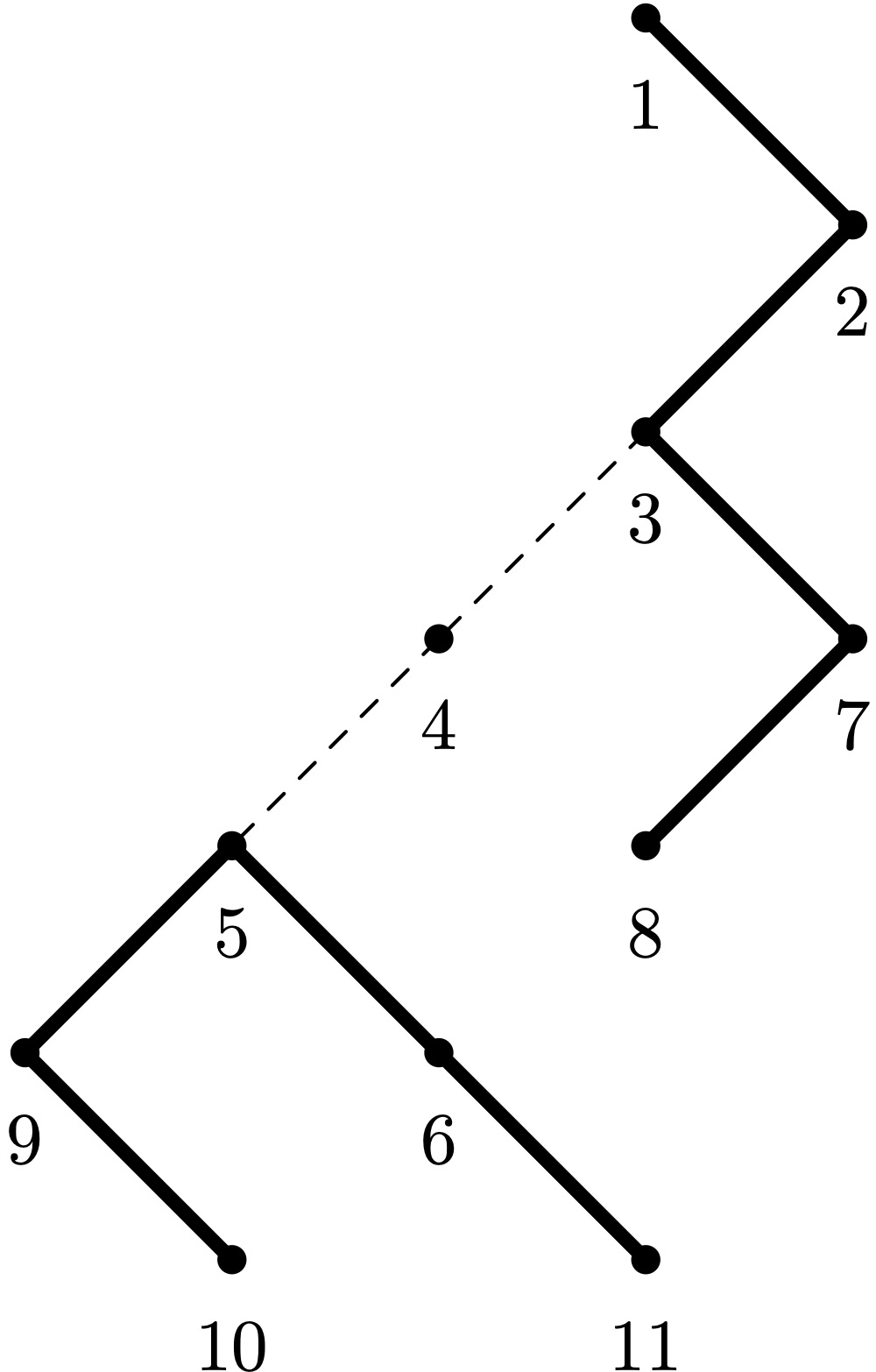시간 제한 메모리 제한 제출 정답 맞은 사람 정답 비율
2 초 256 MB 2 2 2 100.000%

## 문제

You are given an unweighted tree with $n$ vertices, numbered by integers from $1$ to $n$. Let us define the remove operation as follows:

1. choose an arbitrary path in the current graph (a path with only one vertex is valid),
2. remove all vertices along this path, and all edges incident to them.

Calculate the minimum number of such operations to remove all edges. Note that it is allowed to leave some vertices not removed.

## 입력

The first line contains one integer $n$ ($2 \le n \le 2 \cdot 10^5$), the number of vertices in the tree.

The $i$-th of the next $n - 1$ lines contains integers $a_i$ and $b_i$ ($1 \le a_i, b_i \le n$, $a_i \ne b_i$), the numbers of vertices connected by edge $i$.

It is guaranteed that the given graph is a tree.

## 출력

Output one integer: the minimum number of remove operations.

## 예제 입력 1

4
1 2
1 3
1 4


## 예제 출력 1

1


## 예제 입력 2

6
3 4
2 3
5 3
1 3
3 6


## 예제 출력 2

1


## 예제 입력 3

11
1 2
2 3
3 4
4 5
5 6
6 11
5 9
9 10
3 7
7 8


## 예제 출력 3

2


## 힌트

The third example corresponds to the following image: